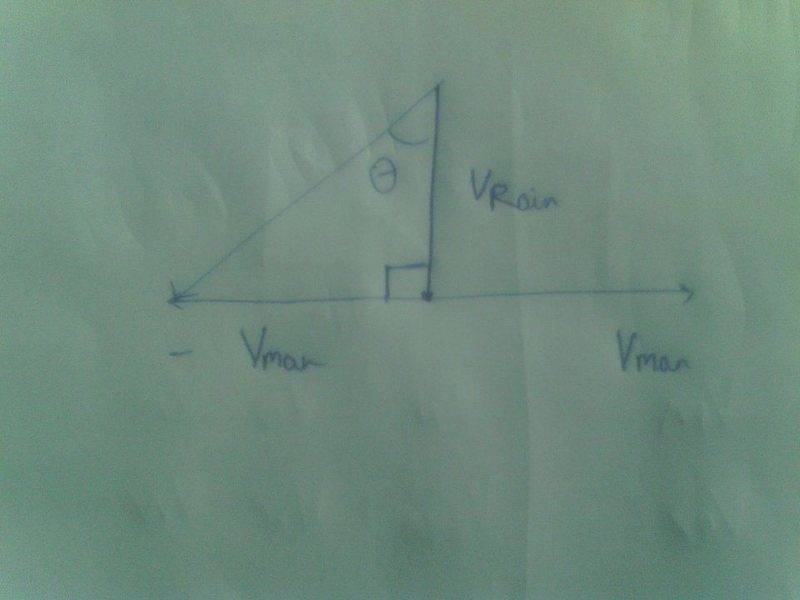# Relative Velocity (Man walking in rain question)

## Homework Statement

A stationary person observes that rain is falling vertically down at 30km/hr. A cyclist is moving on the level road, at 10km/hr. In which direction the cyclist should hold his umbrella to prevent himself from the rain?

2. The attempt at a solution
I used triangle law to find Velocity of rain with respect to man. The angle which comes up is tan^-1 (1/3), but the answer given is tan^-1(3). Where did I go wrong?

Thanks.

I used triangle law to find Velocity of rain with respect to man. The angle which comes up is tan^-1 (1/3), but the answer given is tan^-1(3). Where did I go wrong?

well, when the cyclist is static he will hold the umbrella vertical ; when he is moving he will tilt the umbrella to an angle from the vertical , therefore tan (angle) = perpendicular/base ; so check in your velocity diagram if the answer is , as you are quoting - it must be measuring perpendicular in the rain-velocity vector whose magnitude is 30 km/h then it may be measuring angle from horizontal direction.In your diagram the reverse velocity vector of man will be applied to the rain as well as man separately..
then man will become static and he will observe rain drops which will have two velocities one acting towards left and another vertically downward so resultant will be a velocity completing the upper triangle- now the direction of this resultant making an angle with the horizontal is the direction in which the umbrella could be kept.
In this way one can see how the answer came in to Tan^-1(3). comes up.
Your velocity diagram is to be corrected
If however some body asks -at what angle from vertical direction the umbrella should be kept -then the resultant is making an angle 90- previous calculation angle ......then your answer may have been correct.

•kipup123
Thanks for the help.

•drvrm